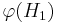# Fully lattice-determined subgroup property

This article defines a subgroup metaproperty: a property that can be evaluated to true/false for any subgroup property
View a complete list of subgroup metaproperties
View subgroup properties satisfying this metaproperty| View subgroup properties dissatisfying this metaproperty
VIEW RELATED: subgroup metaproperty satisfactions| subgroup metaproperty dissatisfactions

## Definition

A subgroup property$p$ is termed fully lattice-determined if, for any lattice-isomorphic groups$G_1, G_2$, any lattice isomorphism$\varphi: L(G_1) \to L(G_2)$, and any subgroup$H_1$ of$G_1$,$H_1$ satisfies$p$ in$G_1$ if and only if$\varphi(H_1)$ satisfies$p$ in$G_2$.

## Relation with other metaproperties

### Weaker metaproperties

Metaproperty Meaning Proof of implication Proof of strictness (reverse implication failure) Intermediate notions
conditionally lattice-determined subgroup property determined up to lattice automorphisms (i.e., holding the ambient group constant) |FULL LIST, MORE INFO